# Text annotations in R with text and mtext functions

## Sample data

The data used in the examples below is the following:

``````# Data
set.seed(123)
x <- 1:50
y <- x + rnorm(50, sd = 10)
groups <- sample(letters, 50, replace = TRUE)``````

## The `text` function

You can add texts to base R plots with the `text` function. You just need to pass the coordinates and the text you want to add.

``````plot(x, y, pch = 19, col = 4)
text(x = 10, y = 50, # Coordinates
label = "Text annotation")``````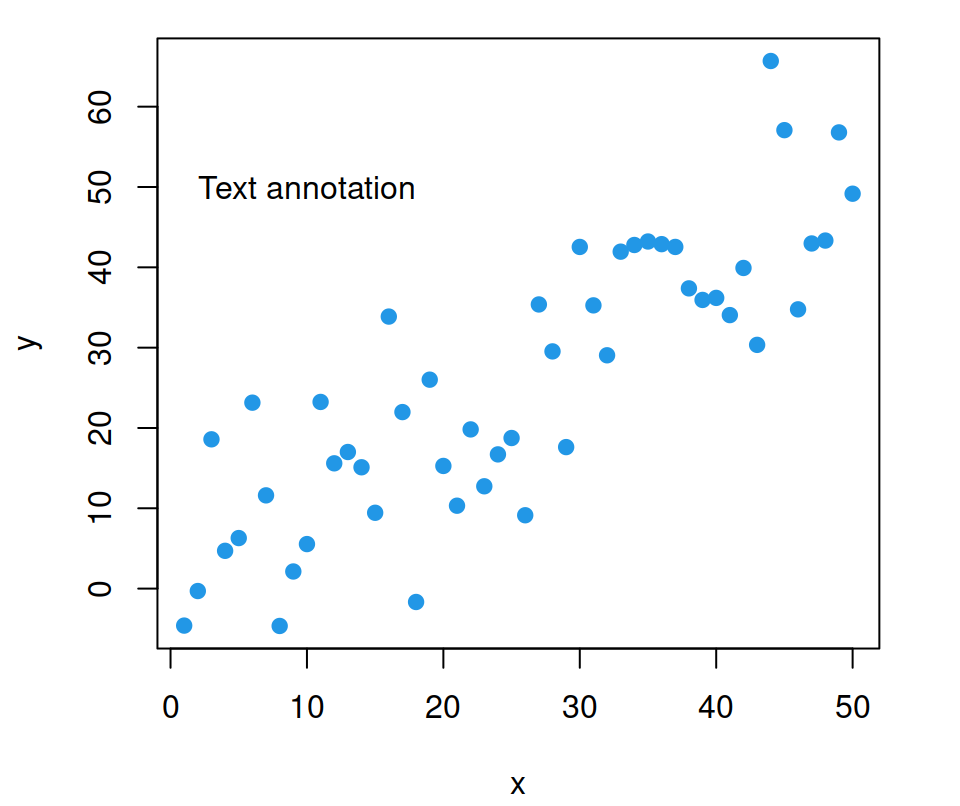Text customization

The added text can be customized with the graphical parameters, such as `col`, for the color, `font` for the font style or `cex` for the text size.

``````plot(x, y, pch = 19, col = 4)
text(x = 15, y = 50, label = "Text annotation",
col = "red",   # Color of the text
font = 2,      # Bold face
cex = 1.5)     # Size``````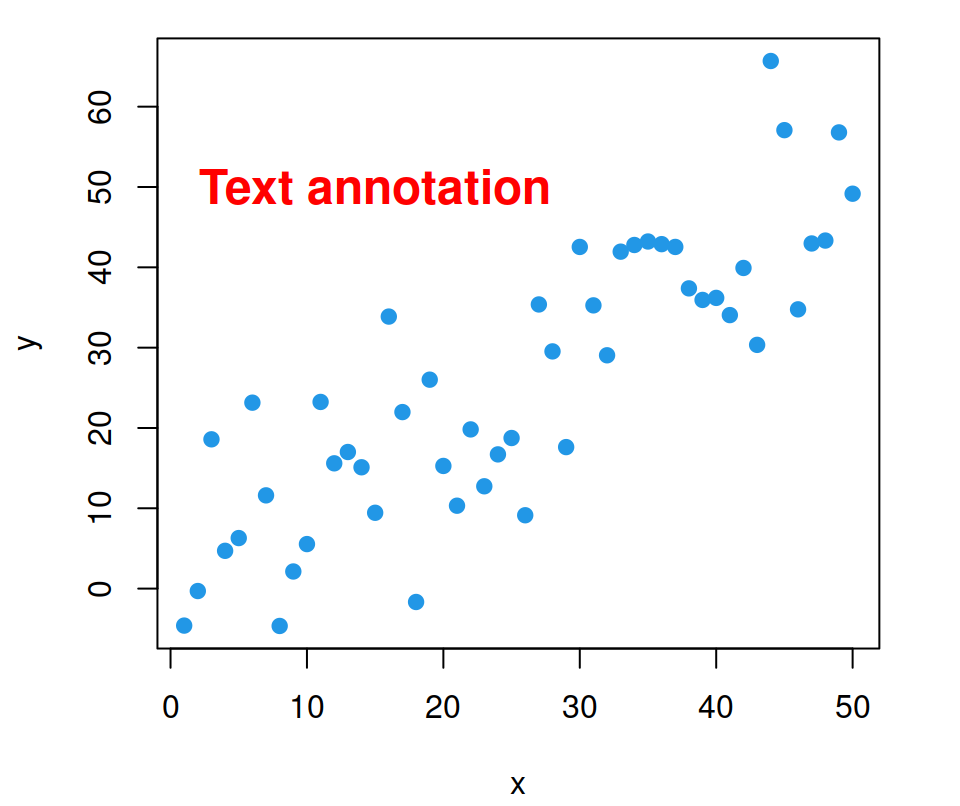Text rotation

You can also rotate the text setting a degree or rotation with the `srt` argument.

``````plot(x, y, pch = 19, col = 4)

# Rotate 45 degrees
text(x = 15, y = 50, label = "Text annotation",
srt = 45) # Rotation``````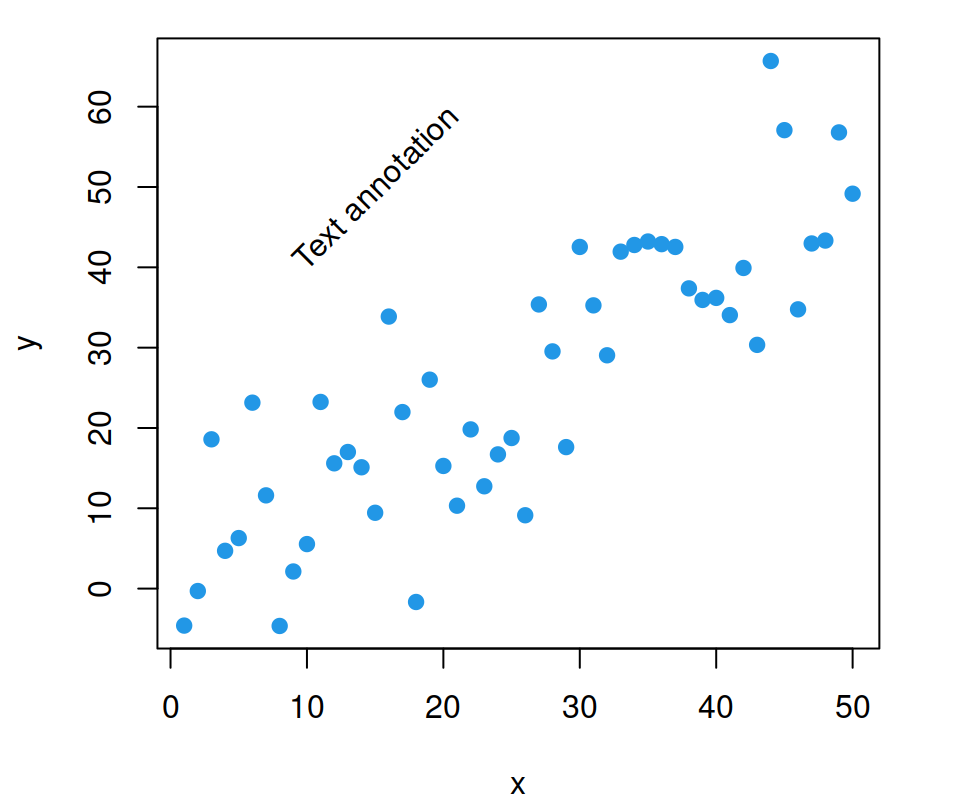Text splitting

Finally, it is worth to mention that you can split the text in several lines with `"\n"`.

``````plot(x, y, pch = 19, col = 4)

# Split the text in several lines
text(x = 15, y = 50,
label = "Text\n annotation") # Split text``````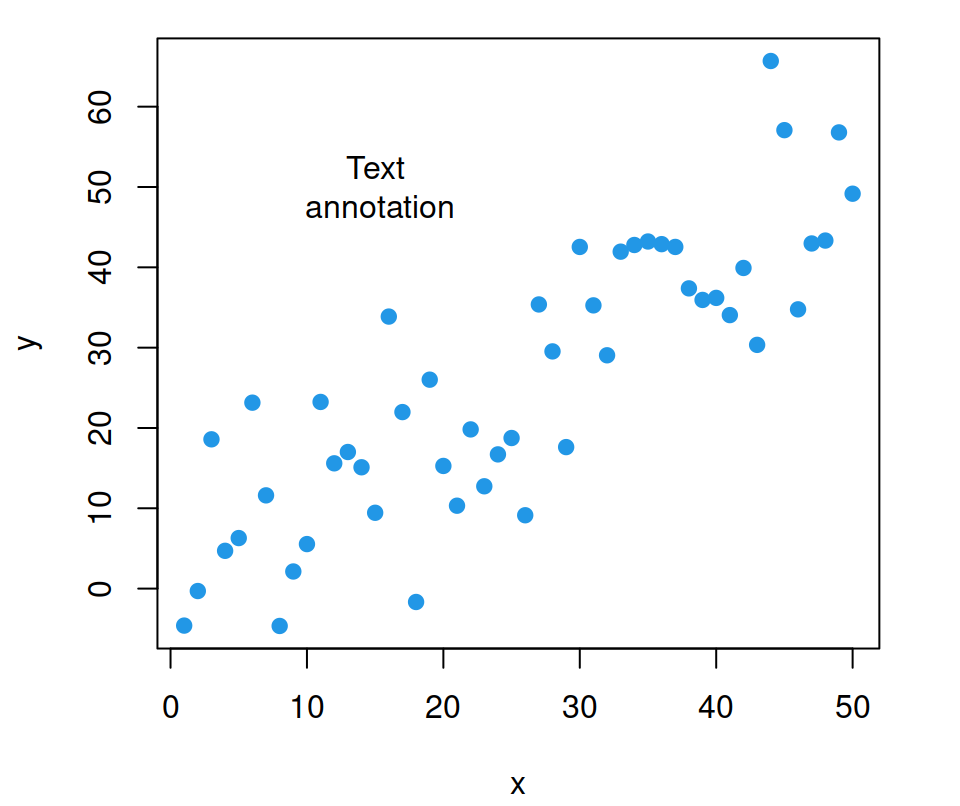### Label points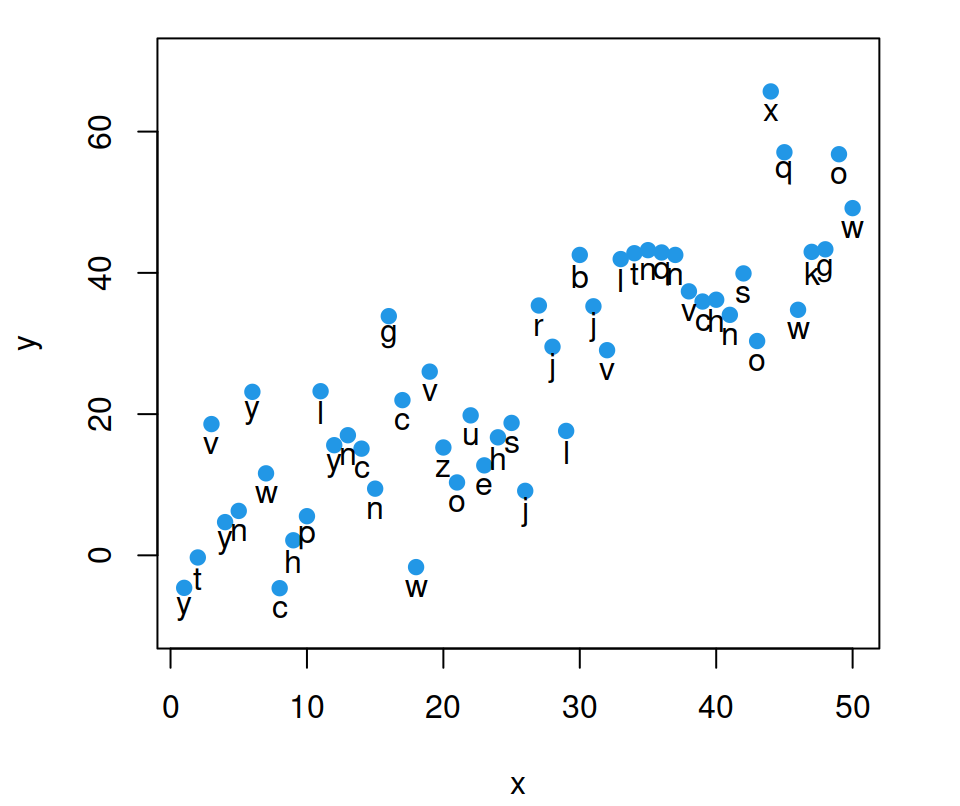If you have a grouping variable you can pass it to the `labels` argument of the `text` function to label the observations. You can set the position specifying the variables and fine-tuning them.

``````plot(x, y, pch = 19, col = 4,
ylim = c(-10, 70)) # Change axis limits
text(x = x, y = y - 3,  # Fine-tune the position
label = groups) ``````

## The `mtext` function

The `mtext` function can be used to add text to all sides of a plot, which can be specified using the `side` argument.

side Position
1 bottom
2 left
3 top
4 right

Bottom text

``````plot(x, y, pch = 19, col = 4)

# Bottom-center
mtext("Bottom text", side = 1)``````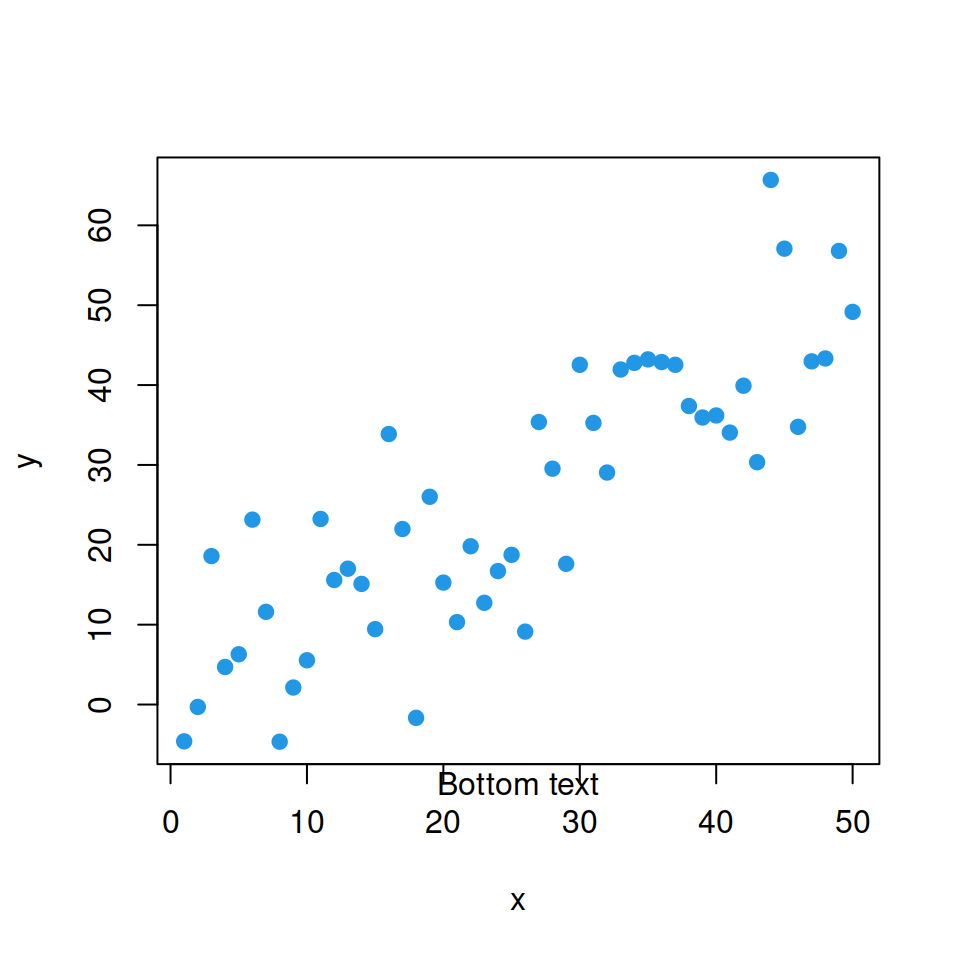Left text

``````plot(x, y, pch = 19, col = 4)

# Left-center
mtext("Left text", side = 2)``````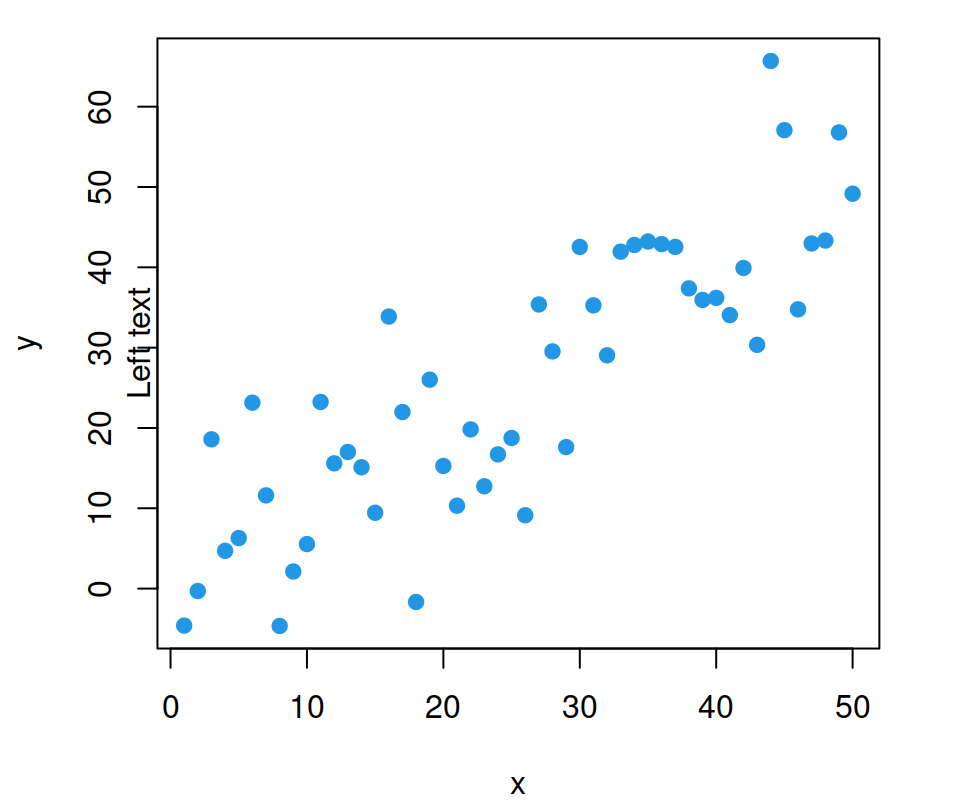Top text

``````plot(x, y, pch = 19, col = 4)

# Top-center
mtext("Top text", side = 3)``````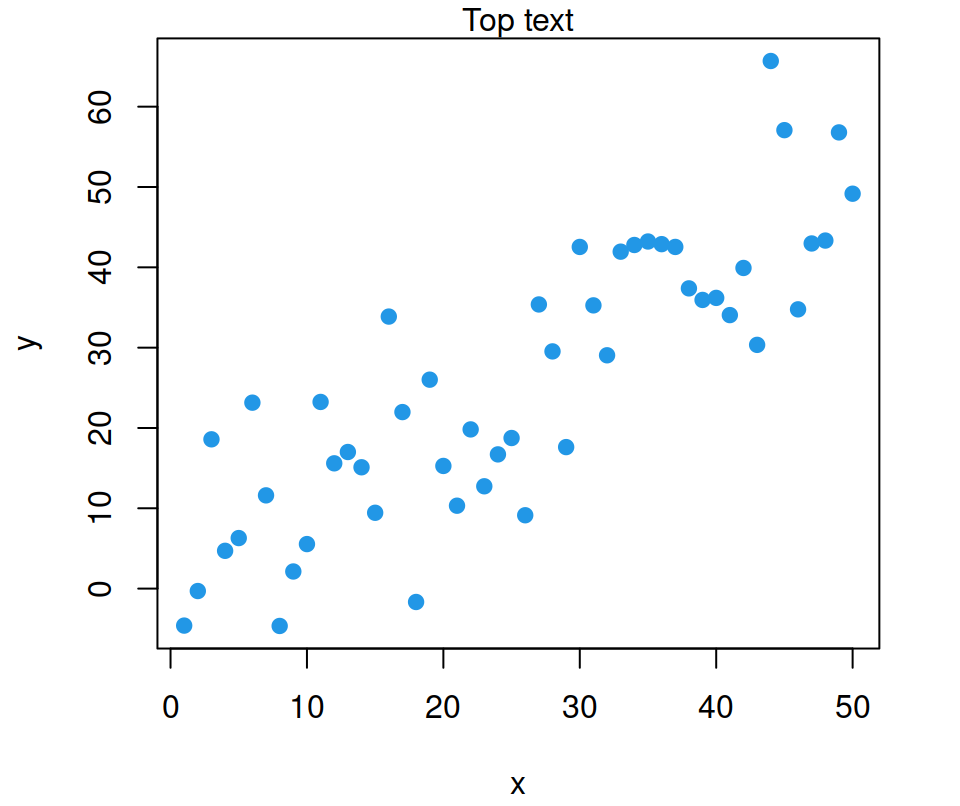Right text

``````plot(x, y, pch = 19, col = 4)

# Right-center
mtext("Right text", side = 4)``````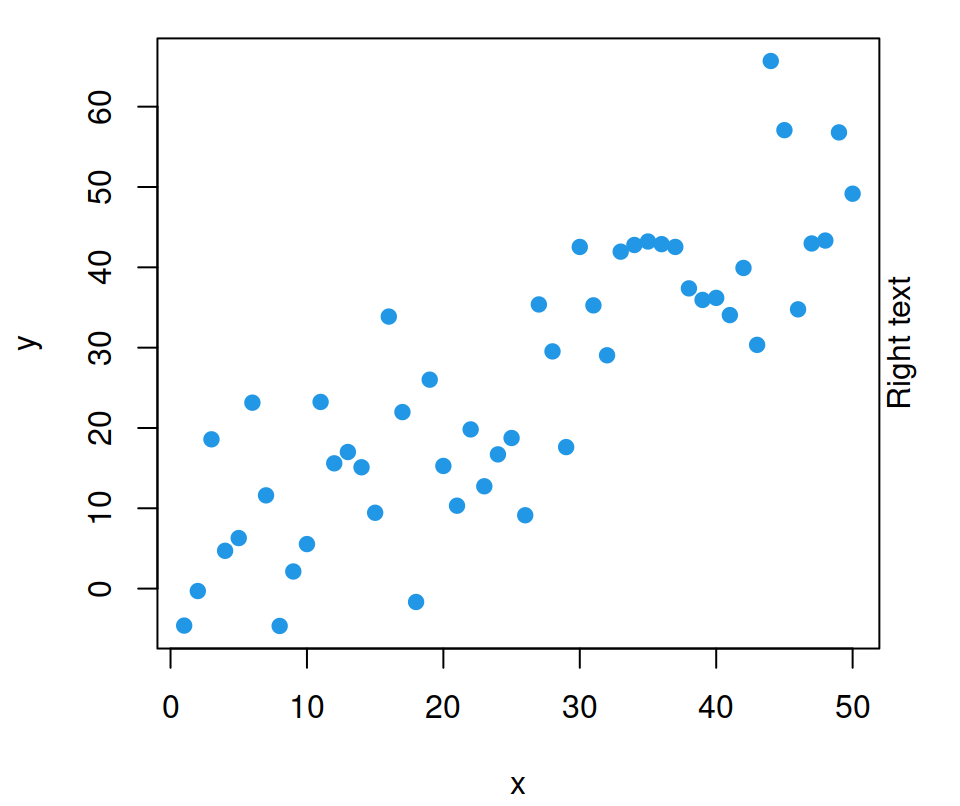### `mtext` adjustment

There are several arguments that you can customize. The most relevant are:

• `line`, to set the margin line where to set the text. Default value is 0.
• `adj`, to adjust the text in the reading direction from 0 to 1 (default value is 0.5).
• `at`, to indicate where to draw the text based on the corresponding axis.
• Other graphical parameters related to texts such as `col`, `cex`, `family` and `font`.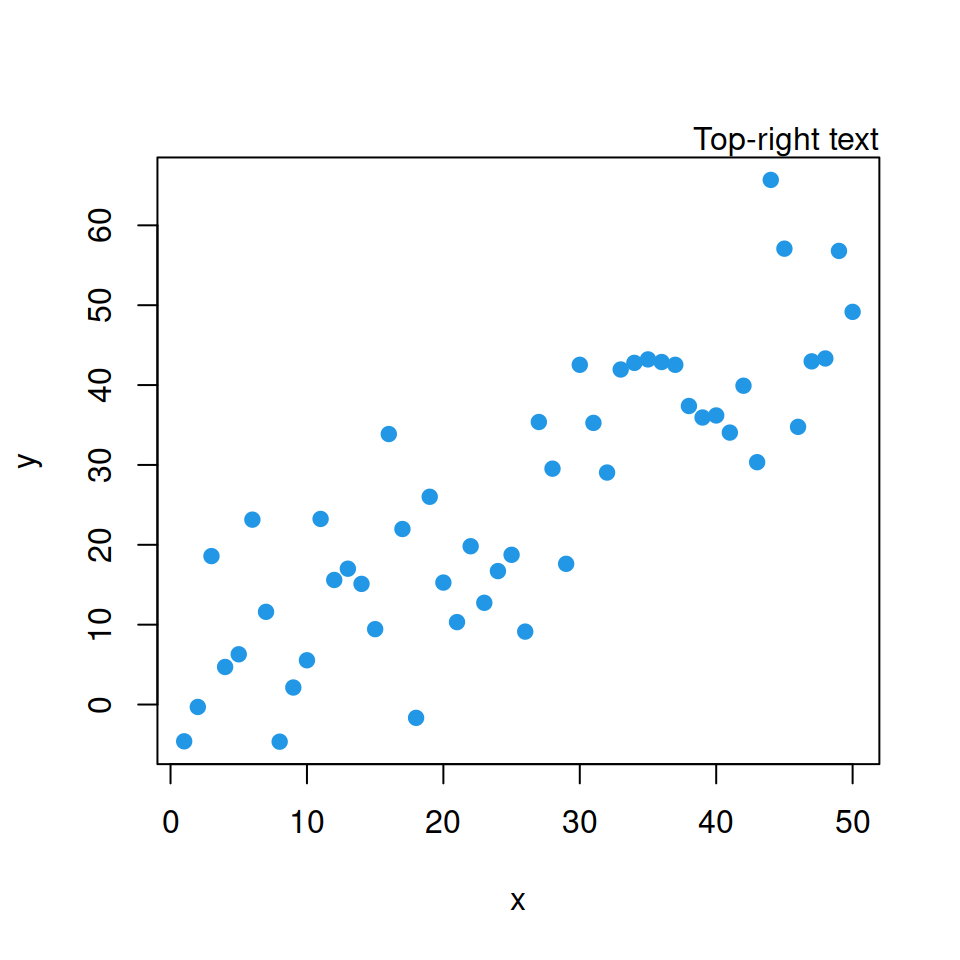Top-right text

``````plot(x, y, pch = 19, col = 4)

# Top-right text
mtext("Top-right text",
side = 3, adj = 1)``````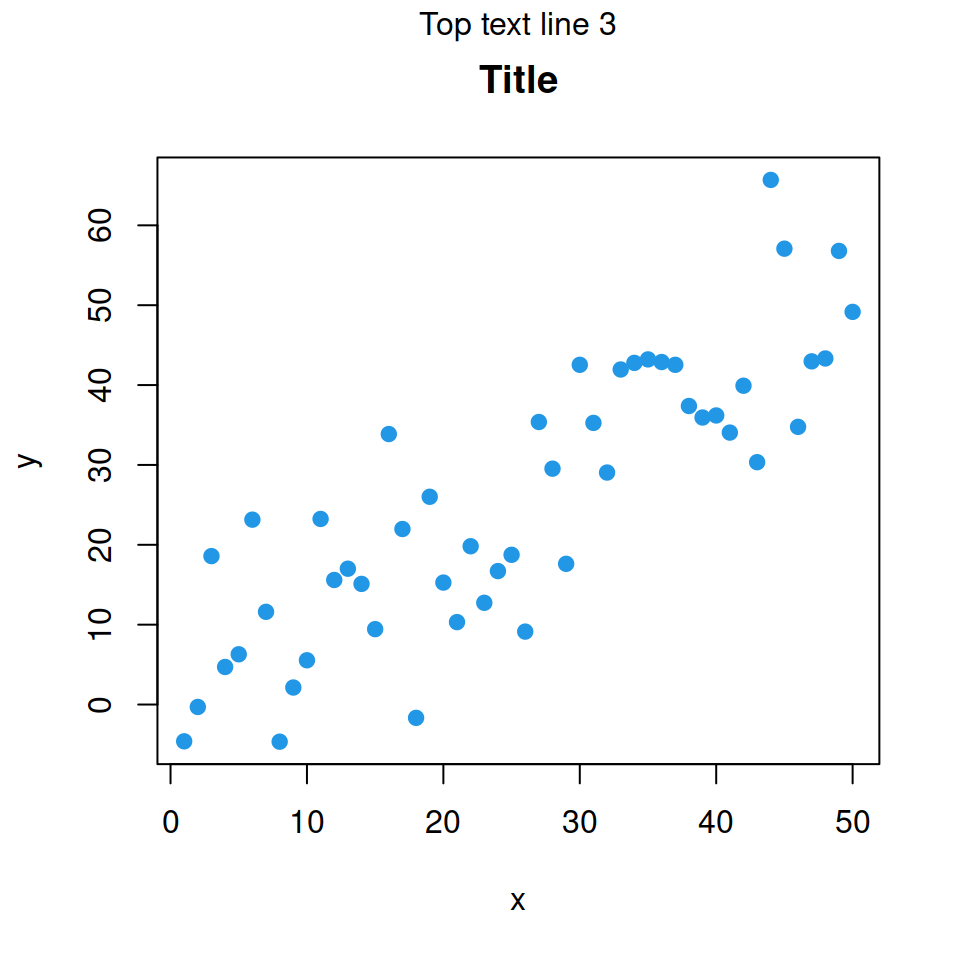Top-center text, line 3

``````plot(x, y, pch = 19, main = "Title", col = 4)

# Top text, line 3 (over the title)
mtext("Top text line 3",
side = 3, line = 3)``````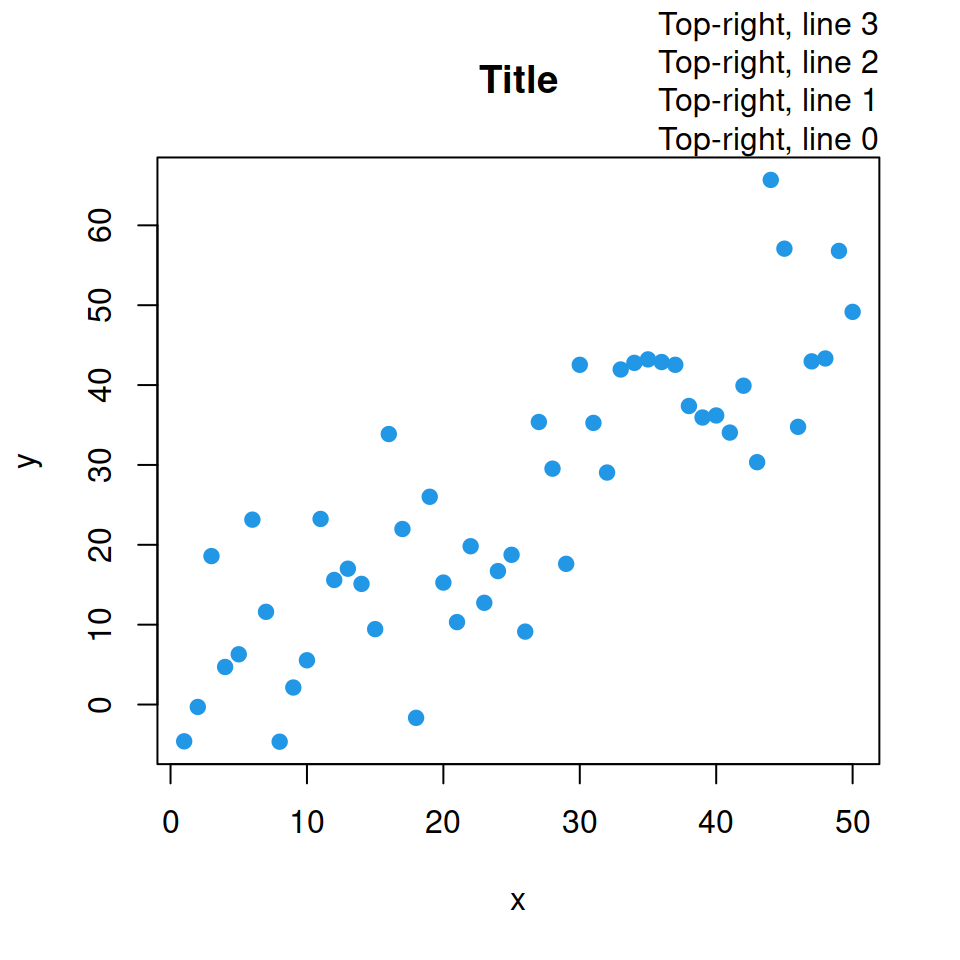Top-right text, lines 0, 1, 2, 3

``````plot(x, y, pch = 19, main = "Title", col = 4)

mtext("Top-right, line 0",
side = 3, adj = 1, line = 0)
mtext("Top-right, line 1",
side = 3, adj = 1, line = 1)
mtext("Top-right, line 2",
side = 3, adj = 1, line = 2)
mtext("Top-right, line 3",
side = 3, adj = 1, line = 3)``````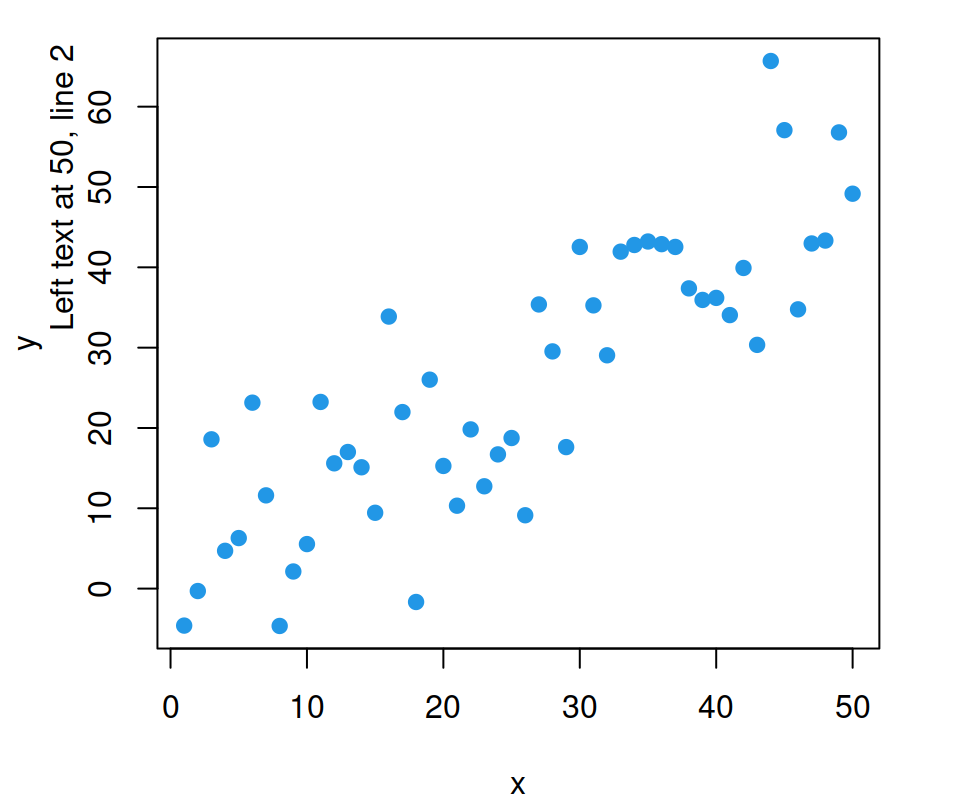Left text at 50, line 2

``````plot(x, y, pch = 19, col = 4)

# Left text, line 2, centered at 50
mtext("Left text at 50, line 2",
side = 2, at = 50, line = 2)``````

`mtext` does not support rotation, only horizontal adjustment with `las = 1` for the vertical axis and vertical adjustment with `las = 3` for the X-axis. If you need to rotate the text you can use `text` function with `srt` argument instead.